Courses

# NCERT Solutions Chapter 15 - Probability, Class 9, Maths Class 9 Notes | EduRev

Created by: Indu Gupta

## Class 9 : NCERT Solutions Chapter 15 - Probability, Class 9, Maths Class 9 Notes | EduRev

The document NCERT Solutions Chapter 15 - Probability, Class 9, Maths Class 9 Notes | EduRev is a part of Class 9 category.
All you need of Class 9 at this link: Class 9

Exercise 15.1

1. In a cricket match, a batswoman hits a boundary 6 times out of 30 balls she plays. Find the probability that she did not hit a boundary.

Total numbers of balls = 30
Numbers of boundary = 6
Numbers of time she didn't hit boundary = 30 - 6 = 24
Probability she did not hit a boundary = 24/30 = 4/5

2. 1500 families with 2 children were selected randomly, and the following data were recorded: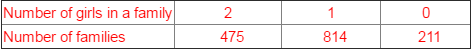Compute the probability of a family, chosen at random, having
(i) 2 girls

(ii) 1 girl

(iii) No girl
Also check whether the sum of these probabilities is 1.

Total numbers of families = 1500
(i) Numbers of families having 2 girls = 475
Probability = Numbers of families having 2 girls/Total numbers of families
= 475/1500 = 19/60
(ii) Numbers of families having 1 girls = 814
Probability = Numbers of families having 1 girls/Total numbers of families
= 814/1500 = 407/750
(iii) Numbers of families having 2 girls = 211
Probability = Numbers of families having 0 girls/Total numbers of families
= 211/1500
Sum of the probability = 19/60 + 407/750 + 211/1500
= (475 + 814 + 211)/1500 = 1500/1500 = 1
Yes, the sum of these probabilities is 1.

3. Refer to Example 5, Section 14.4, Chapter 14. Find the probability that a student of the class was born in August.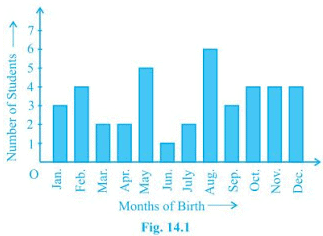Total numbers of students = 40
Numbers of students = 6
Required probability = 6/40 = 3/20

4. Three coins are tossed simultaneously 200 times with the following frequencies of different outcomes: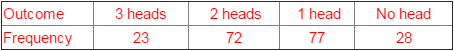If the three coins are simultaneously tossed again, compute the probability of 2 heads coming up.

Number of times 2 heads come up = 72
Total number of times the coins were tossed = 200
Required probability = 72/200 = 9/25

5. An organisation selected 2400 families at random and surveyed them to determine a relationship between income level and the number of vehicles in a family. The information gathered is listed in the table below: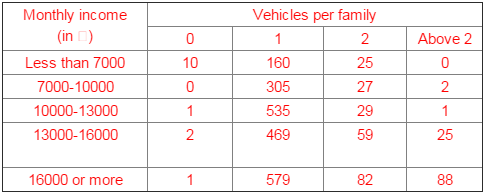Suppose a family is chosen. Find the probability that the family chosen is

(i) earning ₹10000 – 13000 per month and owning exactly 2 vehicles.
(ii) earning ₹16000 or more per month and owning exactly 1 vehicle.
(iii) earning less than ₹7000 per month and does not own any vehicle.
(iv) earning ₹13000 – 16000 per month and owning more than 2 vehicles.
(v) owning not more than 1 vehicle.

Total numbers of families = 2400

(i) Numbers of families earning ₹10000 –13000 per month and owning exactly 2 vehicles = 29
Required probability = 29/2400
(ii) Number of families earning ₹16000 or more per month and owning exactly 1 vehicle = 579
Required probability = 579/2400
(iii) Number of families earning less than ₹7000 per month and does not own any vehicle = 10 Required probability = 10/2400 = 1/240
(iv) Number of families earning ₹13000-16000 per month and owning more than 2 vehicles = 25
Required probability = 25/2400 = 1/96
(v) Number of families owning not more than 1 vehicle = 10+160+0+305+1+535+2+469+1+579 = 2062

Required probability = 2062/2400 = 1031/1200

6. Refer to Table 14.7, Chapter 14.
(i) Find the probability that a student obtained less than 20% in the mathematics test.
(ii) Find the probability that a student obtained marks 60 or above.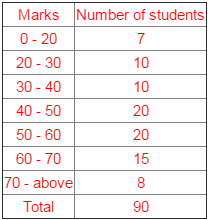Total numbers of students = 90
(i) Numbers of students obtained less than 20% in the mathematics test = 7
Required probability = 7/90
(ii) Numbers of student obtained marks 60 or above = 15+8 = 23
Required probability = 23/90

7. To know the opinion of the students about the subject statistics, a survey of 200 students was conducted. The data is recorded in the following table.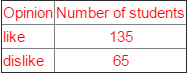Find the probability that a student chosen at random
(i) likes statistics,

(ii) does not like it.

Total numbers of students = 135 + 65 = 200
(i) Numbers of students who like statistics = 135
Required probability = 135/200 = 27/40
(ii) Numbers of students who does not like statistics = 65
Required probability = 65/200 = 13/40

8. Refer to Q.2, Exercise 14.2. What is the empirical probability that an engineer lives:
(i) less than 7 km from her place of work?
(ii) more than or equal to 7 km from her place of work?
(iii) within 1/2 km from her place of work?

The distance (in km) of 40 engineers from their residence to their place of work were found as follows:
5 , 3 , 10 , 20 , 25 , 11 , 13 , 7 , 12 , 31 , 19 , 10 , 12 , 17 , 18 ,  11 , 3 ,  2 ,  17 ,  16 , 2 , 7 , 9 , 7 , 8 ,  3 , 5 , 12 , 15 , 18 , 3 , 12 , 14 , 2 , 9 , 6 , 15 , 15 , 7 , 6 , 12

Total numbers of engineers = 40
(i) Numbers of engineers living less than 7 km from her place of work = 9
Required probability = 9/40
(ii) Numbers of engineers living less than 7 km from her place of work = 40 - 9 = 31
Required probability = 31/40
(iii) Numbers of engineers living less than 7 km from her place of work = 0
Required probability = 0/40 = 0

11. Eleven bags of wheat flour, each marked 5 kg, actually contained the following weights of flour (in kg):
4.97 , 5.05 , 5.08     5.03     5.00     5.06     5.08 , 4.98 ,  5.04 ,  5.07 ,  5.00
Find the probability that any of these bags chosen at random contains more than 5 kg of flour.

Total numbers of bags = 11
Numbers of bags containing more than 5 kg of flour = 7
Required probability = 7/11

12.

In Q.5, Exercise 14.2, you were asked to prepare a frequency distribution table, regarding the concentration of sulphur dioxide in the air in parts per million of a certain city for 30 days. Using this table, find the probability of the concentration of sulphur dioxide in the interval 0.12-0.16 on any of these days.
The data obtained for 30 days is as follows:
0.03 , 0.08 , 0.08 , 0.09 , 0.04 , 0.17 , 0.16 , 0.05 , 0.02 , 0.06 , 0.18 , 0.20 , 0.11 , 0.08 , 0.12 , 0.13 , 0.22 , 0.07 , 0.08 , 0.01 , 0.10 , 0.06 , 0.09 , 0.18 , 0.11 , 0.07 , 0.05 , 0.07 , 0.01 , 0.04

Total numbers of days data recorded = 30 days
Numbers of days in which sulphur dioxide in the interval 0.12-0.16 = 2
Required probability = 2/30 = 1/15

13. In Q.1, Exercise 14.2, you were asked to prepare a frequency distribution table regarding the blood groups of 30 students of a class. Use this table to determine the probability that a student of this class, selected at random, has blood group AB.
The blood groups of 30 students of Class VIII are recorded as follows:
A, B, O, O, AB, O, A, O, B, A, O, B, A, O, O, A, AB, O, A, A, O, O, AB, B, A, O, B, A, B, O.

Total numbers of students = 30
Numbers of students having blood group AB = 3
Required probability = 3/30 = 1/10

Offer running on EduRev: Apply code STAYHOME200 to get INR 200 off on our premium plan EduRev Infinity!

,

,

,

,

,

,

,

,

,

,

,

,

,

,

,

,

,

,

,

,

,

,

,

,

,

,

,

;